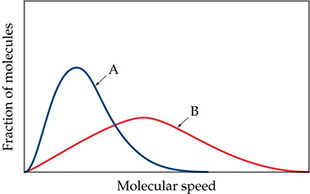# Problem: Consider the following drawing.For each curve, which speed is highest: the most probable speed, the root-mean-square speed, or the average speed?

🤓 Based on our data, we think this question is relevant for Professor Cruz's class at USF.

###### FREE Expert Solution

We are asked which speed is highest: the most probable speed, the root-mean-square speed, or the average speed.###### Problem Details

Consider the following drawing.For each curve, which speed is highest: the most probable speed, the root-mean-square speed, or the average speed?